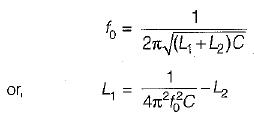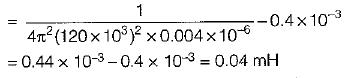Courses

# Signal Generators & Waveform Shaping Circuits

## 10 Questions MCQ Test Topicwise Question Bank for Electronics Engineering | Signal Generators & Waveform Shaping Circuits

Description
This mock test of Signal Generators & Waveform Shaping Circuits for Electronics and Communication Engineering (ECE) helps you for every Electronics and Communication Engineering (ECE) entrance exam. This contains 10 Multiple Choice Questions for Electronics and Communication Engineering (ECE) Signal Generators & Waveform Shaping Circuits (mcq) to study with solutions a complete question bank. The solved questions answers in this Signal Generators & Waveform Shaping Circuits quiz give you a good mix of easy questions and tough questions. Electronics and Communication Engineering (ECE) students definitely take this Signal Generators & Waveform Shaping Circuits exercise for a better result in the exam. You can find other Signal Generators & Waveform Shaping Circuits extra questions, long questions & short questions for Electronics and Communication Engineering (ECE) on EduRev as well by searching above.
QUESTION: 1

### Hysteresis is desirable in Schmitt-trigger because

Solution:

Hysteresis is desirable in a Schmitt trigger because it prevents noise from causing false triggering.

QUESTION: 2

### Assertion (A): All oscillators require positive feedback for their operation. Reason (R): Any circuit which is used to generate a periodic voltage without an a.c. input signal is called an oscillator.

Solution:

Ail oscillators do not require positive feedback for their operation because if the positive resistance of the LC tank circuit is cancelled by introducing the right amount of negative resistance across the tank circuit, then the steady oscillation can be maintained. Some of the devices which possess negative resistance as mentioned above are dynatron, transitron, UJT and tunnel diode. Hence, assertion is not correct.

QUESTION: 3

### Each stage of a three stage amplifier without feedback has identical pole frequencies and its open loop transfer function is expressed as: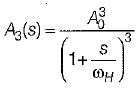What is the maximum value of feedback factor βmax  for which the amplifier remains stable?

Solution: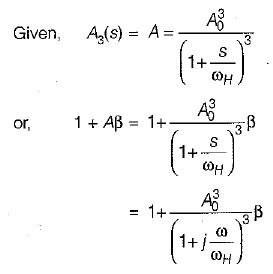The phase change may change Aβ to negative. This can happen only when the total change in the phase angle of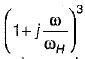becomes 1800 . The  required phase change in one stage i.e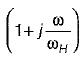should be 600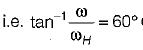or,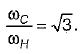∴ Here, ωc is the frequency at which the phase angle changes the sign. The value of Aβ at this frequency is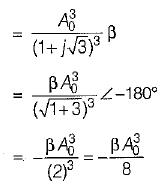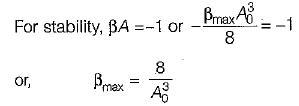QUESTION: 4

Assertion (A): In a crystal oscillator, it is necessary to enclose the crystal in a temperature controlled oven to achieve the frequency stability of the order of 1 partin 1010.
Reason (R): A crystal has very high Q as a resonant circuit, which results in good frequency stability for the oscillator.

Solution:

Both assertion and reason are individually correct statements. The correct reason for the assertion is that the resonant frequency of the crystal is temperature dependent, therefore it is necessary to enclose the crystal in a temperature controlled oven to achieve the required frequeny stability.

QUESTION: 5

Assertion (A): If the magnitude of loop gain of the amplifier is unity then the amplifier gives an a.c. output without any a.c. input signal.
Reason (R): The property of the regenerative feedback is utilised in oscillators.

Solution:

The closed-iooD aain with positive feedback isIf the magnitude of loop gain i.e. |βA| - 1, then Af = ∞. Hence, the gain of the amplifier with positive feedback is infinite and the amplifier gives an a.c. output without any a.c. input signal. Thus, the amplifier acts as an oscillator. Regenerative or positive feedback is utilized in oscillators.
Hence, both assertion and reason are correct but reason is not the correct explanation of assertion.

QUESTION: 6

In a Wien-bridge oscillator, if the value of R is 100 kΩ, and frequency of oscillation is 10 kHz, then the value of capacitance C would be

Solution:

The operating frequency of a Wien-bridge oscillator is given by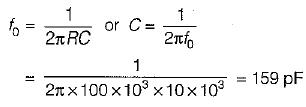QUESTION: 7

A 555 time basically operates in

Solution:
QUESTION: 8

For an RC phase-shift oscillator using BJT, R = 10 kΩ, C = 0.01 μ.F and Rc = 2.2 kΩ The minimum current gain needed for sustained oscillations is around

Solution:

For sustained oscillations, the minimum vaiue of current gain or forward current gain is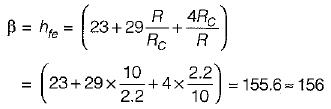QUESTION: 9

A tank circuit contains an inductance of 1 mH. What is the range of tuning capacitor value if the resonant frequency ranges from (540-1650) kHz?

Solution: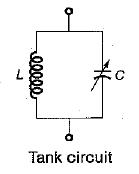For the given tank circuit,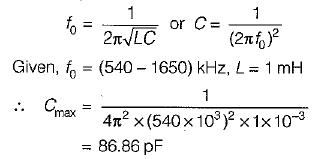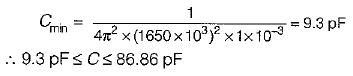QUESTION: 10

ln the Hartley oscillator, L2 = 0.4 mH and C - 0.004 μF. If the frequency of the oscillator is 120 kHz, then the value of L1 would be (neglect the mutual inductance)

Solution:

The frequency of Hartley oscillator is given by